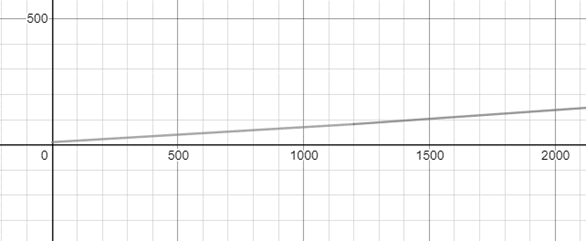# To express: The montly cost as a function of the amount of electricity used and sketch the graph.### Single Variable Calculus: Concepts...

4th Edition
James Stewart
Publisher: Cengage Learning
ISBN: 9781337687805### Single Variable Calculus: Concepts...

4th Edition
James Stewart
Publisher: Cengage Learning
ISBN: 9781337687805

#### Solutions

Chapter 1.1, Problem 60E
To determine

## To express: The montly cost as a function of the amount of electricity used and sketch the graph.

Expert Solution

### Explanation of Solution

Given information:

The base rate of the electricity per month is \$10 , the cost of first 1200kWh uses cost 6cents/kWh and 7cents/kWh for all usage over 1200kWh

The function of the given data is,

E(x)={10+0.06xif0x120010+0.06×1200+0.07(x1200)ifx>1200E(x)={10+0.06xif0x12000.07x2ifx>1200

Thus, the function of the amount of electricity used is E(x)={10+0.06xif0x12000.07x2ifx>1200 .

Consider the graph of the given function,### Have a homework question?

Subscribe to bartleby learn! Ask subject matter experts 30 homework questions each month. Plus, you’ll have access to millions of step-by-step textbook answers!